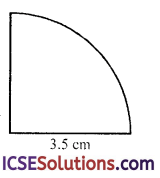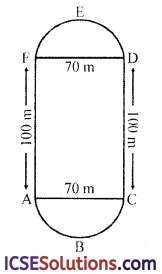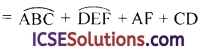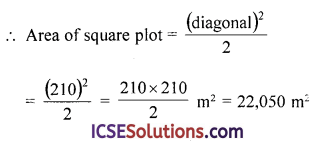The availability of step-by-step S Chand Class 9 Maths Solutions ICSE Chapter 17 Circle: Circumference and Area Ex 17(A) can make challenging problems more manageable.

## S Chand Class 9 ICSE Maths Solutions Chapter 17 Circle: Circumference and Area Ex 17(A)

Take π = $$\frac { 22 }{ 7 }$$ unless stated otherwise.

Question 1.
Find the circumference of the circles whose diameters are :
(i) 49 cm
(ii) 14 cm
(iii) 9.8 cm
(iv) 7 cm
Solution:
(i) Diameter of the circle (d) = 49 cm
∴ Circumference = πd = $$\frac { 22 }{ 7 }$$ × 49 = 154 cm

(ii) Diameter of the circle (d) = 14 cm
∴ Circumference = πd = $$\frac { 22 }{ 7 }$$ × 14 = 44 cm

(iii) Diameter of the circle (d) = 9.8 cm
∴ Circumference = πd = $$\frac { 22 }{ 7 }$$ × 9.8 = 30.8 cm

(iv) Diameter of the circle (d) = 7 cm
∴ Circumference = πd = $$\frac { 22 }{ 7 }$$ × 7 = 22 cmQuestion 2.
Find the circumference of the circles whose radii are :
(i) 7 cm
(ii) 28 cm
(iii) 3.5 cm
(iv) 98 m
Solution:
(i) Radius of the circle (r) = 7 cm
∴ Circumference = 2 πr = 2 × $$\frac { 22 }{ 7 }$$ × 7 = 44 cm

(ii) Radius of the circle (r) = 28 cm
∴ Circumference = 2 πr = 2 × $$\frac { 22 }{ 7 }$$ × 28 = 176 cm

(iii) Radius of the circle (r) = 3.5 cm
∴ Circumference = 2 πr = 2 × $$\frac { 22 }{ 7 }$$ × 3.5 = 22 cm

(iv) Radius of the circle (r) = 98 m
∴ Circumference = 2 πr = 2 × $$\frac { 22 }{ 7 }$$ × 98 = 616 cm

Question 3.
Find the circumference of the circles, if
(ii) Diameter = 15 cm (Take π = 3.14 in (i) and (ii) above.)
Solution:
(i) Radius (r) = 4.5 cm
∴ Circumference = 2 πr = 2 × 3.14 × 4.5 = 28.26 cm = 28.3 cm

(ii) Diameter (d) = 15 cm
∴ Circumference = πd = 3.14 × 15 × cm = 47.10 = 47.1 cm

Question 4.
Find the length of the diameter of the circles whose circumference are :
(i) 22 cm
(ii) 8.8 cm
(iii) 44 cm
Solution:
(i) Circumference = 22 cm
Let d be the diameter of the circle
∴ d = $$\frac{\text { circumference }}{\pi}$$ = $$\frac{\frac{22}{22}}{7}$$ = $$\frac{22 \times 7}{22}$$ = 7 cm
∴ Diameter = 7 cm

(ii) Circumference = 8.8 cm
∴ Diameter = $$\frac{\text { circumference }}{\pi}$$ = $$\frac{\frac{8.8}{22}}{7}$$ = $$\frac{8.8 \times 7}{22}$$ = 0.4 × 7 = 2.8 cm

(iii) Circumference = 44 cm
∴ Diameter = $$\frac{\text { circumference }}{\pi}$$ = $$\frac{\frac{44}{22}}{7}$$ = $$\frac{44 \times 7}{22}$$ = 14 cm

Question 5.
Find the lengths of the radii of the circles whose circumferences are
(i) 11 cm
(ii) 55 cm
(iii) 13.2 m
(iv) 440 m
Solution:
(i) Circumference = 11 cm
Let radius of the circle = r
Then 2πr = 11
⇒ $$\frac{2 \times 22}{7}$$r = 11 ⇒ r = $$\frac{11 \times 7}{2 \times 22}$$ = $$\frac { 7 }{ 4 }$$
∴ Radius = $$\frac { 7 }{ 4 }$$ cm = 1.75 cm

(ii) Circumference = 55 cm
Let radius of the circle = r
Then 2πr = 55
⇒ $$\frac{2 \times 22}{7}$$r = 55 ⇒ r = $$\frac{55 \times 7}{2 \times 22}$$
⇒ r = $$\frac { 35 }{ 4 }$$ = 8.75

(iii) Circumference = 13.2 cm
Let radius of the circle = r
Then 2πr = 13.2 m
⇒ $$\frac{2 \times 22}{7}$$r = 13.2 ⇒ r = $$\frac{13.2 \times 7}{2 \times 22}$$ = $$\frac { 21 }{ 10 }$$ = 2.1

(iv) Circumference = 440 m
Let radius of the circle = r
Then 2πr = 440

⇒ $$\frac{2 \times 22}{7}$$r = 440 ⇒ r = $$\frac{440 \times 7}{2 \times 22}$$ ⇒ r = 70

Question 6.
Find the radii of the circles if circumference is : (π = 3.14)
(i) 100 m
(ii) 6.4 dm
Solution:
(i) Circumference = 100 m
Let radius of the circle = r
∴ 2πr = 100
⇒ 2 × 3.14 × r = 100
⇒ r = $$\frac{100}{2 \times 3.14}$$ = $$\frac{100 \times 100}{2 \times 314}$$
= $$\frac { 10000 }{ 628 }$$ = 15.92 = 15.9 cm

(ii) Circumference = 6.4 dm
Let radius of the circle = r
Then 2 πr = 6.4
⇒ 2 × 3.14 × r = 6.4
⇒ 6.28r = 6.4 ⇒ r = $$\frac{6.4}{6.28}$$
⇒ r = 1.019 = 1.02 dm

Question 7.
The diameter of Venus planet is 12,278 km. Find its circumference.
Solution:
Diameter of Venus planet = 12,278 km
∴Circumference = πd = $$\frac { 22 }{ 7 }$$ × 12278
= 22 × 1754 = 38588 kmQuestion 8.
(i) Find the perimeter of semi-circular plate of radius 3.85 cm.
(ii) The circumference of a circle exceeds the diameter by 16.8 cm. Find the radius of the circle. (Take π = 22/7)
Solution:
(i) Radius of the semicircle = 3.85 cm∴ Perimeter = πr + 2r
= $$\frac { 22 }{ 7 }$$ × 3.85 + 2 × 3.85
= 22 × 0.55 + 7.70
= 12.10 + 7.70 = 19.80 cm

(ii) Let r be the radius of the circle
∴ 2 πr – 2r = 16.8
⇒ 2r (π – 1) = 16.8
⇒ 2r $$\left(\frac{22}{7}-1\right)$$ = 16.8 ⇒ 2r $$\left(\frac{15}{7}\right)$$ = 16.8
⇒ r = $$\frac{16.8 \times 7}{2 \times 15}$$ = $$\frac{168 \times 7}{10 \times 30}$$ = $$\frac{56 \times 7}{100}$$
= $$\frac { 392 }{ 100 }$$ = 3.92
∴Radius of the circle = 3.92 cm

Question 9.
A wire is in form of a circle of radius 42 cm. It is bent into a square. Determine the side of square.
Solution:
Radius of the circular wire = 42 cm
∴ Circumference = 2 πr = 2 × $$\frac { 22 }{ 7 }$$ × 42 cm = 264 cm
On bending it into a square,
The perimeter of square = 264 cm
∴ Side of the square = $$\frac{\text { Perimeter }}{4}$$
= $$\frac { 264 }{ 4 }$$ = 66 cm

Question 10.
In the figure, the radius is 3.5 cm. Find the perimeter of the quarter of the circle correct to one decimal place.Solution:
Cirlce =3.5 cm∴ Perimeter = 2 πr × $$\frac { 1 }{ 4 }$$ + 2r
= $$\frac { 1 }{ 2 }$$ πr + 2r = $$\frac { 1 }{ 2 }$$ × $$\frac { 22 }{ 7 }$$ × 3.5 + 2 × 3.5
= 5.5 + 7.0 = 12.5 cm

Question 11.
(i) The inner circumference of a circular track is 440 m. The track is 14 m wide. Find the diameter of the outer circle of the track. (Take π = 22/7)(ii) The inner edge of a circular running-track is 440 m long. If the track is 10 m wide, find the length of the outer edge of the track.
Solution:
(i) The inner circumference of the circular track = 440 m∴ Radius (r) = $$\frac{\text { circumference }}{2 \pi}$$
= $$\frac{440 \times 7}{2 \times 22}$$ = 70 m

Width of track = 14 m
∴ Radius of the outer circle (R)
= 70 + 14 = 84 m
and diameter = 2R = 2 × 84 = 168 m

(ii) The inner edge of the circular track = 440 m
∴ Inner radius (r) = $$\frac{\text { circumference }}{2 \pi}$$
= $$\frac{440 \times 7}{22 \times 2}$$ = 70 m
Width of track = 10 m
∴ Outer radius (R) = 70 + 10 = 80 m
∴ Length of outer edge (circumference)
= 2πR = 2 × $$\frac { 22 }{ 7 }$$ × 80 = $$\frac { 3520 }{ 7 }$$ m = 502.86 m

Question 12.
A garden roller has a circumference of 3 metres. How many revolutions does it make in moving a distance of 21 metres?
Solution:
Circumference of roller = 3 m
Distance travelled = 21 m
∴ Number of revolutions will be
= $$\frac{\text { Distance }}{\text { circumference }}$$ = $$\frac { 21 }{ 3 }$$ = 7

Question 13.
A bicycle wheel, diameter 70 cm, is making 25 revolutions in 10 sec. At what speed in km/hr is the bicycle travelling?
Solution:
Diameter of the cycle wheel (d) = 70 cm
∴ Circumference = πd = $$\frac { 22 }{ 7 }$$ × 70 = 220 cm
Distance travelled in 25 revolutions
=220 × 25 = 5500 cm = 55 m
∴ Distance of 55 m travelled in = 10 sec.
∴ The distance travelled in 1 hour
= $$\frac { 55 }{ 10 }$$ × 60 × 60 m = 19800 m = 19.8 Km
∴ Speed = 19.8 km/hour

Question 14.
A small cart is being driven at 10 km per hour. If each wheel is 91 cm in diameter, find the number of revolutions made by each wheel per minute.
Solution:
Diameter of wheel (d) = 91 cm
∴ Circumference = πd = $$\frac { 22 }{ 7 }$$ × 91 = 286 cm
Speed = 10 km/hr.
Distance travelled in 1 hour = 10 km
∴ Distance travelled in 1 minute = $$\frac { 10 }{ 60 }$$ Km
= $$\frac{10 \times 1000}{60}$$ m = $$\frac { 500 }{ 3 }$$ m
∴ Number of revolutions in one minute
= $$\frac { 500 }{ 3 }$$ × $$\frac { 100 }{ 286 }$$ (circumference = 286cm)
= $$\frac{500 \times 50}{3 \times 143}$$ = $$\frac { 25000 }{ 429 }$$ = 58$$\frac { 118 }{ 429 }$$

Question 15.
How long will a boy take to go four times round a circular field whose radius is 35 m, walking at 5 km per hour ?
Solution:
Radius of circular field (r) = 35 m
∴ Circumference = 2πr = 2 × $$\frac { 22 }{ 7 }$$ × 35 = 220 m
Distance travelled in 4 rounds = 220 × 4 m = 880 m
Speed of walking = 5km/hour
∴ Time taken to travelled 880 m = $$\frac { 880 }{ 5000 }$$ hr.
= $$\frac{880 \times 60}{5000}$$ = $$\frac { 264 }{ 25 }$$ = 10.56 minute
=10 minute 34 second (approx)

Question 16.
A wheel of a cart is making 4 revolutions per second. If the diameter of the wheel is 84 cm, find its speed.
Solution:
Diameter of the wheel of the cart (d) = 84 cm
∴ Circumference = πd = $$\frac { 22 }{ 7 }$$ × 84 = 264 cm
Distance covered in 1 sec = 4 revolutions
= 4 × 264 = 1056 cm
∴ Distance covered in 1 hour
= 1056 × 60 × 60 cm = $$\frac{1056 \times 60 \times 60}{100 \times 1000}$$ km
= $$\frac { 38016 }{ 1000 }$$ = 38.016 km
∴ Speed of the cart = 38.016 km/hrQuestion 17.
The figure shows a running track in which AF = CD = 100 m and FED and ABC are semicircles whose diameters FD and AC are equal. How much will an athlete run in taking one complete round of the track?Solution:
Diameter of semicircular part = 70 m
∴ Circumference of two semicircular part
= 2 × $$\frac { 1 }{ 2 }$$πd = πd = $$\frac { 22 }{ 7 }$$ × 70 = 220 m
∴ Length of one complete round= 220 + 100 + 100 m = 420 m

Question 18.
A circular field has a perimeter of 660 m. A plot in the shape of a square having its vertices on the circumference is marked in the field, calculate the area of the square plot.
$$\left(\pi=\frac{22}{7}\right) .$$
Solution:
Perimeter (circumference) of the circular field = 660 m∴Length of diagonal of square ABCD
= diameter of the circle = 2r
= 2 × 105 = 210 m